# Bluetooth LE Packet Error Rate Report Integrity Tests

This example shows how to test the packet error rate report integrity of a Bluetooth® low energy (LE) radio frequency (RF) physical layer (PHY) receiver, according to the Bluetooth RF-PHY Test Specification . In this example, you also verify whether these test measurement values are within the limits specified by the Bluetooth RF-PHY Test Specification .

### Objectives of Bluetooth LE RF-PHY Tests

The Bluetooth RF-PHY Test Specification  defined by Bluetooth Special Interest Group (SIG) includes RF-PHY tests for both transmitter and receiver. The objectives of these RF-PHY tests are to:

• Ensure interoperability between all Bluetooth devices

• Ensure a basic level of system performance for all Bluetooth products

Each test case has a specified test procedure and an expected outcome, which the implementation under test (IUT) must meet.

This example deliberately introduces error in the cyclic redundancy check (CRC) field of every alternating packet. The receiver must properly handle the packets with predefined errors. The PER report integrity test validates the error correction mechanism of the receiver. This table shows the test case IDs considered in this example.To simulate the interference tests, perform these steps.

1. Generate test protocol data units (PDUs).

2. Add a 24-bit CRC field by using the `comm.CRCGenerator` object.

3. Corrupt the CRC field in every alternate packet.

4. Generate a Bluetooth LE test waveform by using the `bleWaveformGenerator`.

5. Obtain a passband signal by performing frequency upconversion on the generated test waveform.

6. Scale the passband signal to a desired input level.

8. Downconvert, demodulate, and decode the received signal.

10. Compare the obtained PER with the reference PER and determine the test verdict.

This block diagram summarizes the example workflow.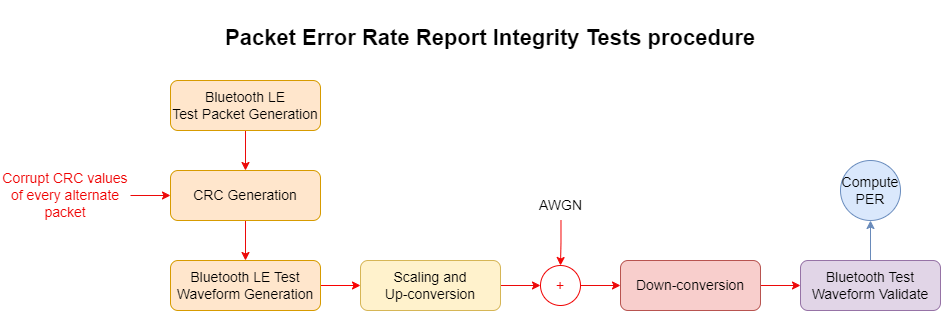### Initialize the Simulation Parameters

Specify the PHY transmission mode, frequency of operation, payload length and samples per symbol. You can adjust the PHY transmission mode and payload length, but the default values in this example are LE1M and 37 bytes, respectively.

```phyMode =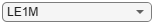'LE1M'; Fc = 2440e6; % Hz payloadLength =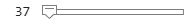37; % Bytes sps = 40; ```

According to the Bluetooth RF-PHY Test Specification , the total number of packets required for testing must be an even number in the range [100, 1500]. Set the number of packets for which the simulation must run.

`packetCount = 50; `

Based on the PHY transmission mode, calculate the sampling rate.

```Rsym = 1e6; if strcmp(phyMode,"LE2M") Rsym = 2e6; end Fs = Rsym*sps;```

### Generate Test Packet PDU

Generate a PRBS9 sequence for the specified payload.

```polynomial = "z^9+z^5+1"; initialConditions = ones(1,9); pnSeq = comm.PNSequence(Polynomial=polynomial, ... InitialConditions=initialConditions, ... SamplesPerFrame=payloadLength*8); payload = pnSeq(); ```

Generate test packet PDU by prepending PDU header and length bits field to payload.

```pduHeader = zeros(8,1); pduLengthBits = int2bit(payloadLength,8,false); % In bits testPacketPDU = [pduHeader; pduLengthBits; payload];```

Generate a synchronization word for the test packet to use as an access address.

`syncWord = flip([1 0 0 1 0 1 0 0 1 0 0 0 0 0 1 0 0 1 1 0 1 1 1 0 1 0 0 0 1 1 1 0].');`

Initialize a CRC generator System object™.

```crcPolynomial = "x^24 + x^10 + x^9 + x^6 + x^4 + x^3 + x + 1"; crcInitValue = "555555"; crcGen = comm.CRCGenerator(crcPolynomial, ... InitialConditions = int2bit(hex2dec(crcInitValue),24)', ... DirectMethod = true); ```

### Simulate PER Report Integrity Test

To perform frequency upconversion, create a digital upconverter System object.

```interpFactor = ceil(2*Fc/Fs)+1; % Interpolation factor upConv = dsp.DigitalUpConverter( ... InterpolationFactor = interpFactor, ... SampleRate = Fs, ... Bandwidth = 2e6, ... StopbandAttenuation = 44, ... PassbandRipple = 0.5, ... CenterFrequency =Fc);```

### Configure Signal Power and Noise

In this simulation the receiver processes a test signal with a specified input level in dBm. Calculate the input signal scaling factor required to generate signal at the desired input level.

```switch phyMode case "LE1M" rxSensitivity =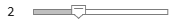-70 % [-70,-104] dBm case "LE2M" rxSensitivity =-70 % [-70,-104] dBm case "LE500K" rxSensitivity =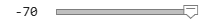-75 % [-70,-108] dBm otherwise % LE125K rxSensitivity =-82 % [-70,-110] dBm end```
```rxSensitivity = -70 ```
```sigLevel = -30; % dBm testInputLevels = rxSensitivity + sigLevel; % dBm scalingFactor = 10^((testInputLevels-30)/20); % Input signal amplitude scaling factor```

Specify noise figure, ambient temperature, bandwidth, and Boltzmann constant values.

```NF = 6; % dB T = 290; % K BW = 2e6; % Hz k = 1.3806e-23; % J/K```

Use thermal noise to simulate the noise floor of the receiver. The height of the noise floor determines the SNR at the receiver. The noise figure of the receiver determines the level of the noise floor. Compute and display the noise floor.

```noiseFloor = 10*log10(k*T*BW)+NF; % dB disp("Receiver noise floor: "+num2str(100+30,"%2.1f")+" dBm")```
```Receiver noise floor: 130.0 dBm ```

To perform frequency downconversion, create a digital downconverter System object.

```downConv = dsp.DigitalDownConverter( ... DecimationFactor = interpFactor, ... SampleRate = Fs*interpFactor, ... Bandwidth = 2e6, ... StopbandAttenuation = 44, ... PassbandRipple = 0.5, ... CenterFrequency = Fc);```

Create an automatic gain control System object.

`agc = comm.AGC(DesiredOutputPower=1);`

Corrupt the CRC value of every alternating packet and append the CRC field to the test packet PDU. Generate a Bluetooth LE test waveform from the test packet PDU by using the `bleWaveformGenerator` function. Upconvert the signal and scale it to the appropriate amplitude level. Pass it through an AWGN channel and downconvert the signal. Decode the downconverted signal and perform packet validation.

```snrdB = -noiseFloor; pktsErr = 0; % Initialize counter for n = 1:packetCount crcErrPos = randi([1 24],1,~rem(n,2)); % CRC error position pduCRC = crcGen(testPacketPDU); % Append CRC % Flip the bit in the CRC based on error position pduCRC(length(testPacketPDU)+crcErrPos) = ~pduCRC(length(testPacketPDU)+crcErrPos); % Generate Bluetooth LE waveform testWaveform = bleWaveformGenerator(pduCRC(:,1),AccessAddress=syncWord,SamplesPerSymbol=sps, ... Mode=phyMode,WhitenStatus="Off"); waveformUp = scalingFactor*upConv([testWaveform;zeros(8*sps,1)]); % Upconvert the signal chanOut = awgn(complex(waveformUp),snrdB,"measured"); % Add thermal noise to the signal downConvOut = downConv(real(waveformUp)); % Perform frequency down conversion agcOut = agc(downConvOut); % Apply AGC [payload,accessAddr] = bleIdealReceiver(agcOut,Mode=phyMode, ... SamplesPerSymbol=sps,WhitenStatus="Off"); % Extract message information [crcFail,pdu] = helperBLETestPacketValidate(payload,accessAddr); % Validate the Bluetooth LE test packet pktsErr = pktsErr + crcFail; end % Determine the PER per = pktsErr/packetCount;```

### Compare Results to Reference Values

Compare the calculated PER value with the reference PER value and display the test verdict.

```[~,refPER] = helperBLEReferenceResults(phyMode,payloadLength); fprintf("Measured PER is %1.4f. For a payload length of %d bytes, this value must be in the range [%1.4f, %1.4f].\n",per,payloadLength,0.5,(0.5+refPER/2));```
```Measured PER is 0.5000. For a payload length of 37 bytes, this value must be in the range [0.5000, 0.6540]. ```
```if per >= 0.5 && per < (0.5+refPER/2) fprintf("PER report integrity test passed.\n"); else fprintf("PER report integrity test failed.\n"); end```
```PER report integrity test passed. ```

### Appendix

This example uses these helper functions:

### Selected Bibliography

1. Bluetooth Special Interest Group (SIG). "Core System Package [Low Energy Controller Volume]". Bluetooth Core Specification. Version 5.3, Volume 6. https://www.bluetooth.com

2. Bluetooth Special Interest Group (SIG). “Bluetooth RF-PHY Test Specification”, RFPHY.TS.p17 Section 4.5. 2022. https://www.bluetooth.com